# Stresses on Octahedral Plane Civil Engineering (CE) Notes | EduRev

## Civil Engineering (CE) : Stresses on Octahedral Plane Civil Engineering (CE) Notes | EduRev

The document Stresses on Octahedral Plane Civil Engineering (CE) Notes | EduRev is a part of the Civil Engineering (CE) Course Advanced Solid Mechanics - Notes, Videos, MCQs & PPTs.
All you need of Civil Engineering (CE) at this link: Civil Engineering (CE)

Stresses on a Octahedral plane
Consider now a tetrahedron element similar to that of figure 4.5 with a plane equally inclined to the principal axes. Hence, its normal is given by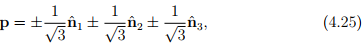where nˆa are the three principal directions of the Cauchy stress tensor. Then, the normal traction, σoct, on this plane is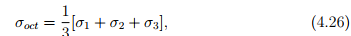obtained using (4.5) and the spectral representation for the Cauchy stress (4.16). Similarly, the shear traction, τoct on this plane is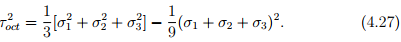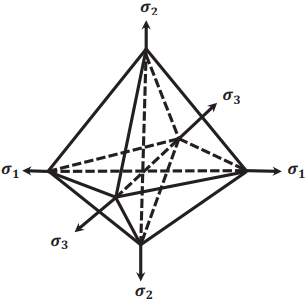Figure 4.5: Eight octahedral planes equally inclined to the principal axes.

From the definition of principal invariants for stress (4.15) it is easy to verify that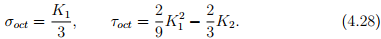Using the above formula one can compute the octahedral normal and shear traction for stress tensor represented using some arbitrary basis.

Offer running on EduRev: Apply code STAYHOME200 to get INR 200 off on our premium plan EduRev Infinity!

## Advanced Solid Mechanics - Notes, Videos, MCQs & PPTs

42 videos|61 docs

,

,

,

,

,

,

,

,

,

,

,

,

,

,

,

,

,

,

,

,

,

;